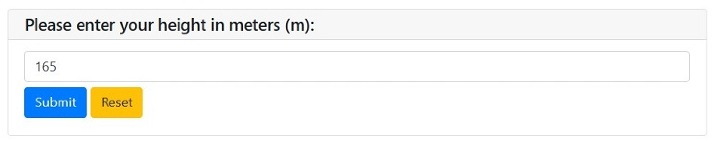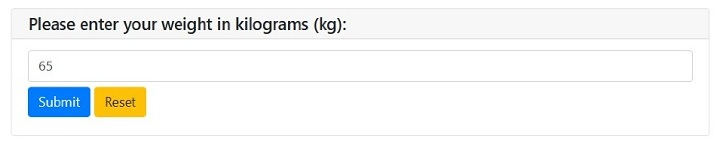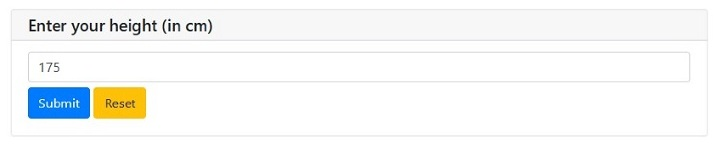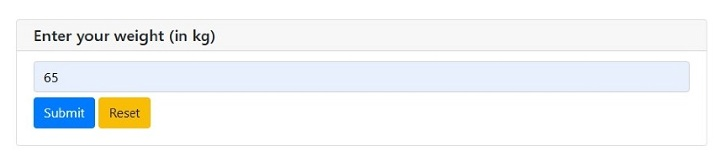# How to create BMI calculator web app using Python and PyWebio?

PyWebio is a python library that can be used to build web applications that require simpler UI. It provides several functions to create a simple web browser. Anyone can build simple web applications using PyWebio without prior knowledge of HTML and JavaScript.

This tutorial will illustrate two methods to create a web to calculate BMI. Body mass index (BMI) measures body fat based on weight and height. It is commonly used to determine whether a person is underweight, normal, overweight, or obese.

## Example

In this example, we define a ‘BMICalculator’ class that contains all the methods needed to calculate and classify BMI. The ‘__init__’ method initializes the object's attributes to None.

Next, we used ‘get_user_inputs()’ method, which gets the user's height and weight using the ‘input()’ function. Then the ‘calculate_bmi()’ method uses the formula to calculate the BMI and rounds the result to two decimal places. The ‘classify_weight_category()’ method uses an if-elif-else statement to classify the user's weight category based on the calculated BMI. The ‘display_results()’ method displays the BMI and weight category to the user using the ‘put_text()’ function.

Finally, we defined the ‘calculate_bmi()’ function that creates an instance of the BMICalculator class, calls its methods in sequence, and displays the results to the user. This function is used as the entry point for the PyWebIO application.

from pywebio.input import input, FLOAT
from pywebio.output import put_text
class BMICalculator:
def __init__(self):
self.height = None
self.weight = None
self.bmi = None
self.classification = None

def calculate_bmi(self):

# Get user's height and weight

# Calculate BMI
self.bmi = self.weight / (self.height ** 2)

# Determine BMI classification
if self.bmi < 16:
self.classification = "Severely underweight"
elif self.bmi < 18.5:
self.classification = "Underweight"
elif self.bmi < 25:
self.classification = "Normal (healthy weight)"
elif self.bmi < 30:
self.classification = "Overweight"
elif self.bmi < 35:
self.classification = "Moderately obese"
else:
self.classification = "Severely obese"

# Display results to the user
put_text("Based on your height of {}m and weight of {}kg, your BMI is {:.1f}. This means you are classified as {}.".format(self.height, self.weight, self.bmi, self.classification))

# Create BMICalculator object
bmi_calculator = BMICalculator()

# Calculate BMI and display results
bmi_calculator.calculate_bmi()


## Output

When you run the above python script, it will open a new window as below −Enter your height in meters and click "Submit" button. On clicking the "Submit" button, it will produce the below screen −Now enter your weight in kilograms and again click the "Submit" button. Upon clicking the "Submit" button, it will show the result as below −

Based on your height of 1.7m and weight of 65kg, your BMI is 22.5. This means you are classified as Normal (healthy weight).


## Example

This is another simple method to create bmi web app. In this example, we defined the ‘calculate_bmi()’ function that prompts users to enter their height and weight. It then calculates the BMI using the formula weight / (height/100) ^2, rounds it to two decimal places, and displays the result using the ‘put_text()’ function. Next, it uses a series of if statements to determine the weight category based on the calculated BMI.

Finally, we used the ‘start_server()’ function to start the web app and display the BMI calculator. We then set the title of the web app to "BMI Calculator" and the text on the "Calculate" button to "Calculate BMI".

from pywebio.input import *
from pywebio.output import *
from pywebio import start_server
def calculate_bmi():
height = input("Enter your height (in cm)", type=FLOAT)
weight = input("Enter your weight (in kg)", type=FLOAT)
bmi = weight / ((height/100) ** 2)
bmi = round(bmi, 2)
weight_category = ""
if bmi < 18.5:
weight_category = "underweight"
elif 18.5 <= bmi <= 24.9:
weight_category = "normal weight"
elif 25 <= bmi <= 29.9:
weight_category = "overweight"
else:
weight_category = "obese"
put_text("Your BMI is: %s" % bmi)
put_text("You have a %s" % weight_category)
if __name__ == '__main__':
start_server(calculate_bmi, port=80, debug=True, title="BMI Calculator", button_text="Calculate BMI")


## Output

When you run the above python script, it will open a new window as below −Enter your height in meters and click "Submit" button. On clicking the "Submit" button, it will produce the below screen −Now enter your weight in kilograms and again click the "Submit" button. Upon clicking the "Submit" button, it will show the result as below −

Your BMI is: 21.22
You have a normal weight


We learned that Pywebio is a powerful library for creating simple web applications. Developers can easily make web applications that require simpler ui. It provides input/output functions that handle the conversion between Python variables and web page elements, making it easy to build interactive web interfaces. One of the key advantages of PyWebIO is its ease of use. We can start quickly by installing the library and importing the necessary functions into your Python code. PyWebIO also provides a wide range of built-in widgets, such as text boxes, drop-down menus, and buttons, which can be easily incorporated into web applications. It supports multiple web frameworks, including Flask, Django, and Tornado, which makes it easy to integrate with existing Python web applications

Updated on: 10-Apr-2023

316 Views